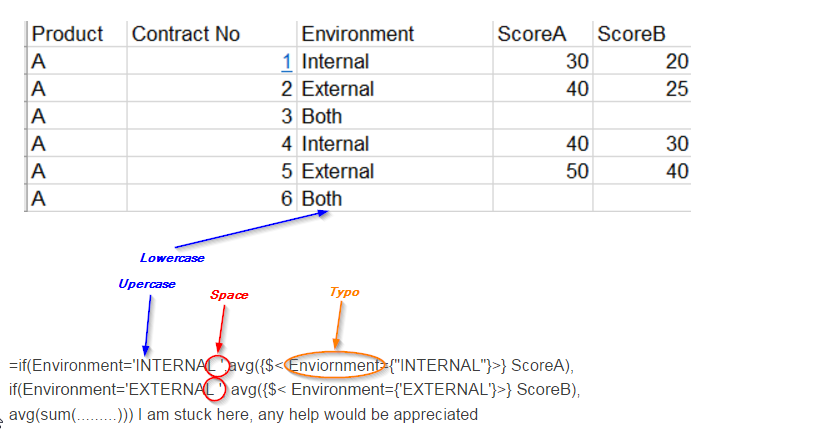# QlikView Creating Analytics

Discussion Board for collaboration related to Creating Analytics for QlikView.

Highlighted
Valued Contributor

## How to sum 2 fields in an IF statementIf Environ is Internal, I want the avg of Score A, If env is External, I want Avg of ScoreB, If its both, I want the avg of

scoreA and scoreB. how to write the IF statement as an expn in Straight Table.

=if(Environment='INTERNAL ',avg({\$< Enviornment={"INTERNAL"}>} ScoreA),

if(Environment='EXTERNAL ', avg({\$< Environment={'EXTERNAL'}>} ScoreB),

avg(sum(.........))) I am stuck here, any help would be appreciated

Tags (3)
1 Solution

Accepted SolutionsMVP

## Re: How to sum 2 fields in an IF statement

=if(Environment='INTERNAL ',avg({\$< Enviornment={"INTERNAL"}>} ScoreA),

if(Environment='EXTERNAL ', avg({\$< Environment={'EXTERNAL'}>} ScoreB),

RangeAvg( avg({\$< Enviornment={"INTERNAL"}>} ScoreA),avg({\$< Environment={'EXTERNAL'}>} ScoreB))

))

9 RepliesMVP

## Re: How to sum 2 fields in an IF statement

As per above example, what is the value of ScoreA average.. ScoreB average and what is ScoreAandB both average..MVP

## Re: How to sum 2 fields in an IF statement

Finding it difficult to understand what is your expected output from the given sample?MVP

## Re: How to sum 2 fields in an IF statement

Maybe using Rangesum()?

avg( Rangesum(ScoreA, ScoreB))

Valued Contributor

## Re: How to sum 2 fields in an IF statement

if it is Internal env, it shoud give me an avg for (30+40) which is 35. If it is external, it should avg (25+40) which is 32.5;

if it is both it should avg the averages just caculated, which means it sud avg (35+32.5) which is 33.75MVP

## Re: How to sum 2 fields in an IF statement

May be this:

=If(Environment='INTERNAL ', Avg(TOTAL <Product> {\$< Enviornment={"INTERNAL"}>} ScoreA),

If(Environment='EXTERNAL ', Avg(TOTAL <Product>{\$< Environment={'EXTERNAL'}>} ScoreB),

RangeAvg(Avg(TOTAL <Product> {\$< Enviornment={"INTERNAL"}>} ScoreA), Avg(TOTAL <Product>{\$< Environment={'EXTERNAL'}>} ScoreB))MVP

## Re: How to sum 2 fields in an IF statement

=if(Environment='INTERNAL ',avg({\$< Enviornment={"INTERNAL"}>} ScoreA),

if(Environment='EXTERNAL ', avg({\$< Environment={'EXTERNAL'}>} ScoreB),

RangeAvg( avg({\$< Enviornment={"INTERNAL"}>} ScoreA),avg({\$< Environment={'EXTERNAL'}>} ScoreB))

))

Valued Contributor

## Re: How to sum 2 fields in an IF statement

Swuehl,

Thank you so much. It looks like your soln is correct. Let me plug in the changes in my qvw and I will get back to close this thread.

regards,

D

Contributor

## Re: How to sum 2 fields in an IF statement

swuehl, solution will work.

just another way to implement, which i read in one of the blogs works better

=pick(match(Environment,'INTERNAL','EXTERNAL','BOTH')

avg({\$< Enviornment={"INTERNAL"}>} ScoreA),

avg({\$< Environment={'EXTERNAL'}>} ScoreB),

RangeAvg( avg({\$< Enviornment={"INTERNAL"}>} ScoreA),avg({\$< Environment={'EXTERNAL'}>} ScoreB))

)Partner

## Re: How to sum 2 fields in an IF statement

Hi QlikDash,

Sunny and Swuehl give you the correct answer. They copied and completed your expression, but your expression was not correctTheir response with corrections:

=If(Environment='Internal', Avg(TOTAL <Product> {\$< Environment={'Internal'}>} ScoreA),

If(Environment='External', Avg(TOTAL <Product>{\$< Environment={'External'}>} ScoreB),

RangeAvg(Avg(TOTAL <Product> {\$< Environment={'Internal'}>} ScoreA), Avg(TOTAL <Product>{\$< Environment={'External'}>} ScoreB))

))